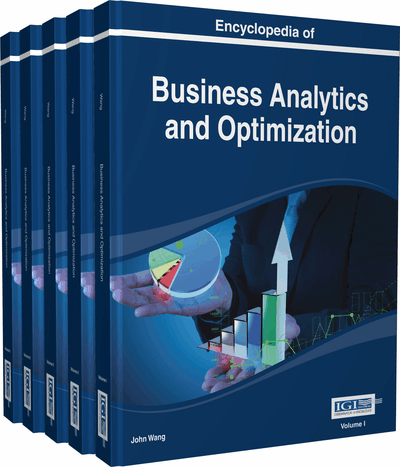# Global Induction of Classification and Regression Trees

Marek Kretowski (Bialystok University of Technology, Poland) and Marcin Czajkowski (Bialystok University of Technology, Poland)
DOI: 10.4018/978-1-4666-5202-6.ch098
Available
\$29.50
No Current Special Offers

Top

## Introduction

Decision trees are one of the most widely used prediction techniques in data mining (Fayyad, Piatetsky-Shapiro, Smyth, & Uthurusamy, 1996). Their similarity to a human reasoning process makes them popular not only among data analysts but also business users. Tree-based approaches are easy to understand, visualize, interpret and their output model can be explained in typical business terms. The ease of application, fast operation and what may be the most important, i.e. the effectiveness of the decision trees, makes them powerful and popular tool (Kotsiantis, 2013).

Two main types of decision trees’ approaches can be distinguished by the type of the problem they are applied to. Tree predictors can be used to classify existing data (classification trees) or to approximate real-valued functions (regression trees) (see Figure 1). In each leaf, classification tree assigns a class label (usually the majority class of all instances that reach that particular leaf), while the regression tree holds a constant value (usually an average value for the target attribute). In addition, model tree is an extension of regression tree (see Figure 1). Main difference between regression tree and model tree is that, for the latter, constant value in the terminal node is replaced by the regression function. Each leaf of the model tree holds a linear (or nonlinear) model whose output is the final prediction value.

Figure 1.

An example of univariate decision tree with tests on nominal and continuous-valued features. Depending on the tree type, leaves could contain class (classification tree), constant value (regression tree) or some kind of model (model tree).

Most typical tree-based system applies greedy search in decision tree induction. One major drawback of greedy algorithms is that, they search only for locally best splits at each node which does not guarantee the globally best solution. Hence, applications of Evolutionary Algorithms (EAs) to the problem of decision tree induction become increasingly popular alternative. Instead of local search, EAs perform a global search in the space of candidate solutions.

The purpose of this chapter is to illustrate the application of EAs to the problem of decision tree induction. The objectives are to show that evolutionary optimization, compared to the greedy search, may result in finding globally optimal solutions, whose complexity is significantly smaller and the prediction is highly competitive. We will cover the global induction of classification, regression, and model trees.

Top

## Background

Inducing optimal decision tree is known to be NP-complete (Naumov, 1991). Consequently, practical decision-tree learning algorithms are based on heuristics such as greedy algorithms where locally optimal splits are made in each node. The most popular tree-induction is based on the top-down approach (Rokach & Maimon, 2005). Top-down induction starts from the root node, where locally best split (test) is searched, according to the given optimality measure (e.g. Gini, towing or entropy rule for classification tree and least squared or least absolute deviation error criterion for regression tree). Next, the training instances are redirected to the newly created nodes and this process is repeated for each node until some stopping-rule is satisfied. The recursive partitioning of the dataset may lead to the data over-fitting, therefore, the decision tree pruning (Esposito, Malerba, & Semeraro, 1997) is applied to improve the generalization power of the predictor.

Most of tree inducing algorithms partition the feature space with axis-parallel hyper-planes. These types of trees are called univariate decision trees. Split at each non-terminal node usually involves single feature. For a continuous-valued feature usually an inequality test with binary outcomes is applied and for a nominal attribute mutually exclusive groups of attribute values are associated with outcomes. One of the first and most well-known solution that can be applied to classification and regression problem is CART (Breiman, Friedman, Olshen, & Stone, 1984) system. Good representatives of univariate inducers are also systems developed by Quinlan: C4.5 (1993) for classification and M5 (1992) for regression.

## Key Terms in this Chapter

Decision Tree Pruning: An approach that reduces the size of decision tree by removing parts of the tree that provide little power to classify/predict the data. This technique improves the generalization power of the decision tree and reduces the over-fitting.

Model Tree: An extension of regression trees were the constant value in the terminal node is replaced by the regression plane.

Decision Tree: A decision tree is a graph that uses a branching method to illustrate every possible outcome of a decision. Each branch of the decision tree represents a possible decision or occurrence. The tree structure shows how one choice leads to the next, and the use of branches indicates that each option is mutually exclusive.

Global Induction: A method of decision tree generation, where both the tree structure and all tests are searched at the same time; usually based on evolutionary approach in contrast to top-down induction.

Top-Down Induction: A recursive method of decision tree generation. It starts with the entire input dataset in the root node where a locally optimal test for data splitting is searched and branches corresponding to the test outcomes are created. The test searches and data splitting are repeated in the created nodes unless the stopping condition is met.

Over-Fitting: The problem existing in supervised learning when a classifier or regressor perfectly predicts the training data but performs much worse on unseen, testing set. Problem can emerge when machine learning algorithm fits noise or insufficient data, i.e., when it learns on irrelevant facts or generalizes from specific cases.

Multivariate Decision Tree: A decision tree with tests involving several attributes. The most common form of such tests is an oblique split, which is based on a linear combination of features (hyper-plane).

Univariate Decision Trees: A decision tree which partitions the feature space with axis-parallel hyper-planes. Each split at non-terminal node involves single feature.

## Complete Chapter List

Search this Book:
Reset